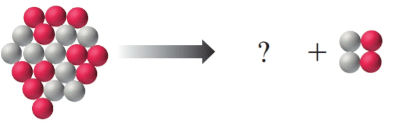# The figure below represents the decay of Na-20 by alpha emission. Count the protons (red) and neutrons (gray), and draw in the missing particle. Write a nuclear equation to represent this decay.### Chemistry In Focus

7th Edition
Tro + 1 other
Publisher: Cengage Learning,
ISBN: 9781337399692

#### Solutions

Chapter
Section### Chemistry In Focus

7th Edition
Tro + 1 other
Publisher: Cengage Learning,
ISBN: 9781337399692
Chapter 8, Problem 69E
Textbook Problem
1 views

## The figure below represents the decay of Na-20 by alpha emission. Count the protons (red) and neutrons (gray), and draw in the missing particle. Write a nuclear equation to represent this decay.Interpretation Introduction

Interpretation:

The missing particle of the nuclear reaction is to be identified.

Concept Introduction:

Radioactivity is the spontaneous and continual disintegration of the unstable atomic nucleus.

There are three types of radiation emitted by the disintegration of the unstable nucleus, such as alpha, beta, and gamma radiation.

### Explanation of Solution

Alpha decay is the type of radioactive decay in which the unstable atomic nucleus dissipates energy by ejecting an alpha particle. An alpha particle consists of two protons and two neutrons.

The number of red bolls on the reactant side is 11, so, the proton count will be 11. The number of grey bolls on the reactant side is 9, so, the neutron count will be 9. The atomic number of the atom is the number of protons in the nucleus. The atom which has the atomic number 11 is sodium. The atomic mass number is the total number of protons and neutrons in the nucleus. The atomic mass of the atom is 20. The nuclear equation must be balanced. The number of neutrons and protons on both sides of the equation must be equal. The missing particle would contain 9 red bolls and 7 grey bolls. The atom which has the atomic number 9 is a fluorine atom...

### Still sussing out bartleby?

Check out a sample textbook solution.

See a sample solution

#### The Solution to Your Study Problems

Bartleby provides explanations to thousands of textbook problems written by our experts, many with advanced degrees!

Get Started

Find more solutions based on key concepts
Which two minerals are the major constituents of bone? a. calcium and zinc b. sodium and magnesium c. phosphoru...

Nutrition: Concepts and Controversies - Standalone book (MindTap Course List)

Hemiacetals are sometimes referred to as potential aldehydes. Explain.

Chemistry for Today: General, Organic, and Biochemistry

Where should the police car in Figure 6-9 have parked to make a good measurement?

Horizons: Exploring the Universe (MindTap Course List)

What is the function of the central vacuole in plants?

Biology: The Dynamic Science (MindTap Course List)

What is hereditarianism, and what is the invalid assumption it makes?

Human Heredity: Principles and Issues (MindTap Course List)

Water is pumped up from the Colorado River to supply Grand Canyon Village, located on the rim of the canyon. Th...

Physics for Scientists and Engineers, Technology Update (No access codes included)